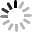Events

# Thermal Imaging•DRI

March 6, 2023When a customer decides to buy an infrared camera, how far it can see is a very important and very hard to explain question.

In order to understand how to calculate this distance, we need to know the Johnson criteria first.

The Johnson Criteria is the standard that is used for DRI (Detection, Recognition, and Identification). It is calculated based on how many pixels are necessary in order to make an accurate evaluation of your object.

Detection

Detection is defined as: if a target is found in the field of view, the image of the target must account for more than 1.5 pixels in the critical dimension direction

Recognition

Recognition is defined as: the target can be classified to identify whether the target is a car, truck or person, which means that the image of the target must occupy more than 6 pixels in the critical dimension direction

Identification

The definition of recognition is that the model and other characteristics of the target can be distinguished. For example, to distinguish between the enemy and us, the image of the target must occupy more than 12 pixels in the critical dimension direction

The above data are obtained under the condition that the probability is 50%, that is, the target can be just found, and the contrast between the target and the background is 1. From the Johnson criterion above, it can be seen that how far an infrared thermal imager can see is determined by the target size, lens focal length, detector performance and other factors.Factors that determine DRI range

1. Lens focal length

The most important factor that determines the detection distance of an infrared camera is the lens focal length. It directly determines the size of the image formed by the target, that is, the number of pixels on the focal plane. This is usually expressed in terms of spatial resolution. It represents the angle of each pixel opening in the space of the object, that is, the minimum angle that the system can resolve. It is generally derived from the ratio of pixel size (d) to focal length (f), that is, IFOV=d/f

The image of each target in the focal plane occupies several pixels, which can be calculated from the target size, the distance between the target and the thermal imager, and the spatial resolution (IFOV). The ratio of the target size (D) and the distance (L) between the target and the thermal imager is the target angle, and then divided by IFOV to get the number of pixels occupied by the image, that is, n=(D/L)/IFOV=(DF)/(LD). It can be seen that the larger the focal length, the more pixels the target image occupies. According to Johnson's criterion, its detection distance is longer. On the other hand, the larger focal length the lens has, the smaller the angle of view is, and the higher the cost will be.

For example, if the pixel size of the focal plane of thermal camera is 38um and equipped with a 100mm focal length lens, the spatial resolution IFOV is 0.38mrad. Observe the target with the size of 3.2m in 1km, and the open angle of the target is 2.3mrad. The image of the target occupies 2.3/0.38=6 pixels. According to Johnson's criterion, the recognition level is reached

2. Infrared Detector performance

The lens focal length determines the detection distance of thermal imaging camera theoretically. Another factor that plays an important role in practical applications is the thermal imaging detector performance. The lens focal length only determines the image size and the number of pixels occupied, while the thermal imaging sensor performance determines the image quality, such as the blurring degree and the signal-to-noise ratio.

3. Atmospheric environment

Although the penetration of thermal radiation to the atmosphere is stronger than that of visible light, the absorption and scattering of the atmosphere still have a certain impact on the image quality of thermal imager. Especially in some harsh weather environments like heavy fog and heavy rain, the detection distance of infrared thermal imager will be affected.

--------------------------------------------------------------------